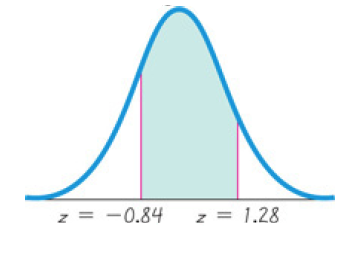×
Get Full Access to Elementary Statistics - 12 Edition - Chapter 6.2 - Problem 11bsc
Get Full Access to Elementary Statistics - 12 Edition - Chapter 6.2 - Problem 11bsc

×

# Answer: Standard Normal Distribution. In Exercises, findISBN: 9780321836960 18

## Solution for problem 11BSC Chapter 6.2

Elementary Statistics | 12th Edition

• Textbook Solutions
• 2901 Step-by-step solutions solved by professors and subject experts
• Get 24/7 help from StudySoup virtual teaching assistantsElementary Statistics | 12th Edition

4 5 1 359 Reviews
13
1
Problem 11BSC

Standard Normal Distribution. In Exercises 9–12, find the area of the shaded region. The graph depicts the standard normal distribution of bone density scores with mean 0 and standard deviation 1.Equation Transcription:Text Transcription:

z=-0.84

z=1.28

Step-by-Step Solution:
Step 1 of 3

Problem 11BSC

Step1 of 2:

We have In Exercises, find the area of the shaded region. The graph depicts the standard normal distribution of bone density scores with mean 0 and standard deviation 1.Step2 of 2:

From the above graph we have P(p < z <q ).

P(p < z <q ) = P(-0.84 < z < 1.28)

= P(z < q) - P(z < p)

= P(z < 1.28) - P(z < -0.84)

area under normal curve can be calculated by using standard normal table or statistical table that is P(z < 1.28) = 0.8997 (i.e, row 1.2 under column 0.08)

P(z < -0.84) = 0.2005 (i.e, row -0.8 under column 0.04)

consider,

P(-0.84 < z < 1.28) = P(z < 1.28) - P(z < -0.84)

= 0.8997 - 0.2005

= 0.6992.

Therefore, the area of the shaded region is 0.6992.

Step 2 of 3

Step 3 of 3

##### ISBN: 9780321836960

Since the solution to 11BSC from 6.2 chapter was answered, more than 538 students have viewed the full step-by-step answer. This textbook survival guide was created for the textbook: Elementary Statistics, edition: 12. Elementary Statistics was written by and is associated to the ISBN: 9780321836960. The answer to “?Standard Normal Distribution. In Exercises 9–12, find the area of the shaded region. The graph depicts the standard normal distribution of bone density scores with mean 0 and standard deviation 1. Equation Transcription:Text Transcription: z=-0.84z=1.28” is broken down into a number of easy to follow steps, and 35 words. This full solution covers the following key subjects: standard, normal, distribution, Find, deviation. This expansive textbook survival guide covers 121 chapters, and 3629 solutions. The full step-by-step solution to problem: 11BSC from chapter: 6.2 was answered by , our top Statistics solution expert on 03/15/17, 10:30PM.

Unlock Textbook Solution